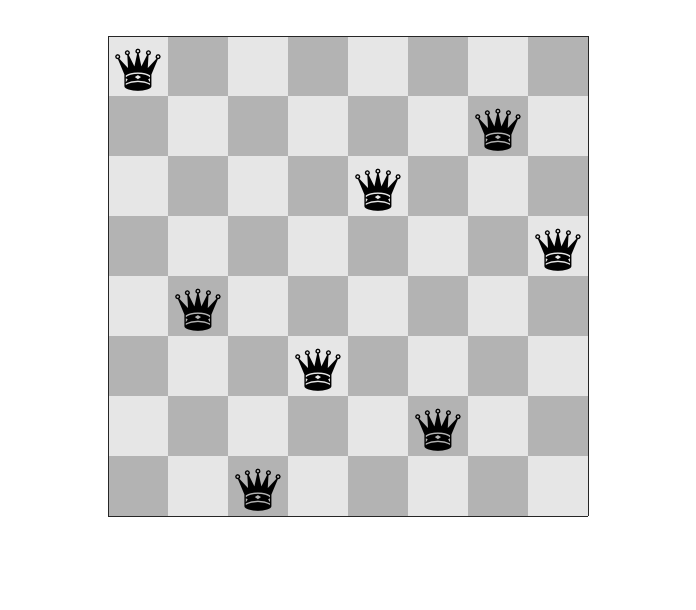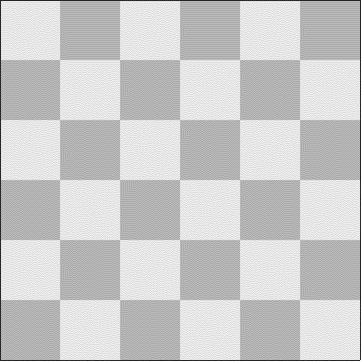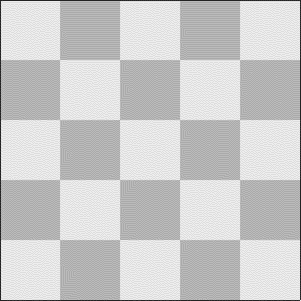# The Eight Queens Problem

Today I want to tackle a classic algorithm puzzle known as the Eight Queens Problem. In this problem, your task is to arrange eight queens on a chessboard so that none of the queens is attacking any of the others. Here is one solution.The problem is often restated as the N Queens Problem, which is placing N queens on an N-by-N board.

This is more of a general algorithm topic than an image processing topic, although some of the concepts and MATLAB techniques that I show might be useful to people doing image processing work. For some reason, this problem has been on my mind recently, so I decided to play with it a bit in MATLAB. I ended up with both a working algorithm and an interesting visualization. Here is the result for a 6x6 chessboard.Solving the eight queens problem was the first "real" and nontrivial program that I can recall writing. I wrote it in Basic for a math class project sometime around 1981. My school didn't actually have a computer at that point; I used a teletype terminal (no monitor!) and a dial-up modem to communicate with the county school system's mainframe, which was located some miles away. As I recall, the program used a pure brute-force solution, generating boards randomly and testing each one to see if it was a valid solution. I knew almost nothing about algorithms then.

When I revisited the problem this week, I used a recursive divide-and-conquer technique with backtracking. I'll explain that in two parts, starting with the recursive divide-and-conquer part. The most famous algorithm in signal processing, the fast Fourier transform (FFT) is based on this idea. The discrete Fourier transform of an $N$-point sequence can be written in terms of the discrete Fourier transforms of two different subsets of the original sequence.

Here is one way to use divide-and-conquer on the N queens problem. We'll write a routine that places N queens on an N-column board in two steps:

A. Place one queen on a safe square somewhere on the first column of the board.

B. Call the routine recursively to place N-1 queens on an (N-1)-column board.

The backtracking part is necessary because, unlike when computing the FFT, one of the substeps A or B might fail.

If step B fails, then we backtrack. That is, we undo step A, find a different safe square on the first column of the board, and then do the recursive step B call again. At any time, if there are no remaining safe squares on the first column to try, then the solution attempt has failed and the routine returns.

Here's some MATLAB code to implement this idea.

function [board,succeeded] = placeQueens(board,col)
N = size(board,1);
if col > N
% There are no columns left. All the queens
% have been placed. Return with success.
succeeded = true;
return
end
safe = false(1,N);
for row = 1:N
safe(row) = isSafe(board,row,col);
end
if ~any(safe)
% There are no safe squares on this column.
% Return with failure.
succeeded = false;
return
end
for row = 1:N
if safe(row)
board(row,col) = 1;
[board,succeeded] = placeQueens(board,col+1);
if succeeded
return
else
% Backtrack. Undo the placement of the queen
% on this column and keep looking.
board(row,col) = 0;
end
end
end
% If we get here, we have checked every row on this column
% without succeeding. Return with failure.
succeeded = false;

While I was generating the animations for boards of different sizes, two things caught my eye. First, there's no solution for a 6x6 board that has a queen in any corner. Look at the animated solution above and see if you can convince yourself about that.

Second, I noticed that a solution for the 5x5 board can be found without any backtracking. Check out the animation:Next time, I'll write about the graphics programming and GIF and video file writing I did to do the algorithm visualization.

Published with MATLAB® R2017a

|